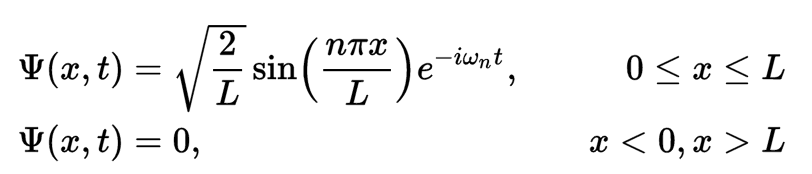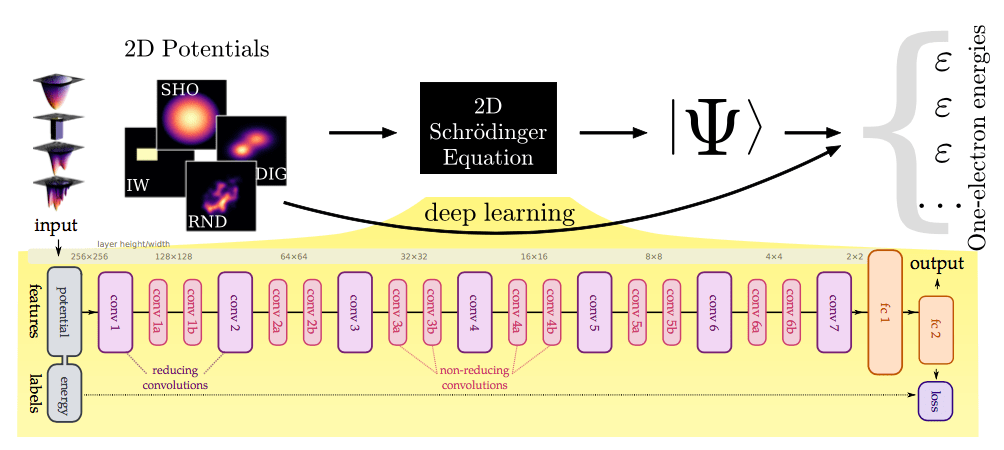# Hey Computer, What If I Don’t Want to Solve my Schrödinger Equation?

Compared to simplifying or approximating the solution of a complicated equation, deep learning provides a highly flexible model and might serve as a perfect walk-around method.

In the past year, dozens of research papers on deep learning application in physics were published on arXiv. Here are some of the well-known examples:

The last one was even recommended by Yann LeCun as an “unexpected application of ConvNets”. In this article, I will try to break down the complicated physics background, and explain the paper from a software engineer perspective for those who do not have much knowledge about physics. I will also introduce several discussions I had with people in the area of physics, and demonstrate the tremendous potential of applying deep learning in computational physics.

Before we dive into the details, we need to first reach a consensus about the meaning of “understanding” an substance. In the definition of physics, understanding a substance means we are able to predict the status of that substance at any arbitrary time. Physicists further summarized that it is equivalent to knowing two attributes of the substance: its current status, and its movement. This is corresponding to the wave function of a particle in the world of quantum physics, which is exactly what the Schrödinger equation tries to describe. Once we solved Schrödinger equation, we would be able to get the wave function of the particle and calculate its position or energy (so that we understand the past, current and future of this particle). However, we are very much limited by the capability of computation, and physicists have been using approximation and simplification to accomplish this task, much like what we did in the past using linear or mixture of Gaussian in the area of machine learning. This method will inevitably run into the problem that it describes the real world poorly.

Let’s stop here and go back to our initial goal. What we want to know in the first place is the status of the substance at any arbitrary time. Schrödinger equation (or the wave function derived from it) is merely one of the many techniques (yet a very powerful one) to answer this question. Let’s carefully avoid understanding the meaning of the equation and take a look at some wave function examples that can be solved from Schrödinger equation:

1.     Free particle2.    Infinite potential wellWe find that these are essentially very simple natural exponential functions of position and time.

So what if we do not solve Schrödinger equation (nor even understand it), but instead train a blind end-to-end neural network to give us the attributes of the particle we care about? Maybe using electrostatic potentials to predict the ground-state energy of an electron? The answer is yes, and that is what this paper is about.

The paper uses a 19-subsequent-convolution-layer model as the architecture of the network. It takes an input of a two-dimensional potential, represented as a 256×256 grey-scale image, and outputs one electron’s energy (Є, Є, …, Є).FIG 1. Network architecture used in Deep learning and the Schrodinger equation

The paper uses mean-squared error(MSE) between the true energy and the convolution neural network prediction as the loss function, and AdaDelta optimizer in the training. The work validates that the ground-state energy of an electron in four classes of confining two-dimensional electrostatic potentials can be predicted via this architecture with acceptable accuracy, especially the case of randomly generated potentials, where there is no analytic form for either the potential or the ground state energy.

At this point of time, it is not too optimistic to assume that the above network captures the wave function of the electron. So how about predict the wave function itself? Maybe that could greatly accelerate our understanding for this physical world.

Furthermore, if what professor Henry Lin and Max Tegmark pointed out in paper Why does deep and cheap learning work so well? is solid, similar techniques might be applied to other areas of physics. A friend of mine who is passionate about fluid mechanics once told me, the governing equation, Navier–Stokes equation, is impossible to solve (at least up to this point), and one of their main research topics is raising reasonable assumptions to simplify the analysis. Given fluid mechanics has an abundance of experimental observations and simulation results, the idea of introducing deep learning method sounds very plausible.

The predecessor of quantum mechanics, Dirac, once said “…approximate, practical methods of applying quantum mechanics should be developed, which can lead to an explanation of the main features of complex atomic systems without too much computation.” Compared to simplifying or approximating the solution of a complicated equation, deep learning provides a highly flexible model and might serve as a perfect walk-around method. We dare to imagine the landscape of computational physics, which occupies one third of modern physics, will be completely change in the near future.

Thanks to Qianchi Feng, Hao Yu for providing the physics explanation. This article could not be completed without your helps.

Analyst: Yiran Mao |Editor: Hao Wang|Localized by Synced Global Team : Xiang Chen